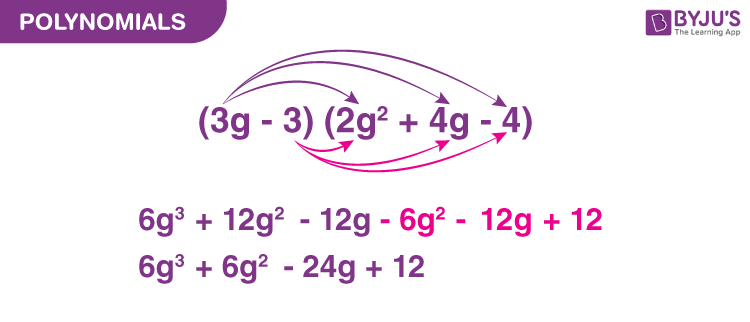# Polynomials Worksheets

Polynomials Worksheets are designed with a vision to improve the mathematical skills of the students who are weak in the Polynomials chapter. These worksheets cover all the basic concepts of Polynomials for the CBSE class 9 students.

You will study polynomials, math formulas, and the fundamental expressions of algebra through the math worksheets. We use polynomials to represent quantities in general, such as perimeter, area, revenue, and the volume of blood flowing through the artery.Every year, the CBSE or the central board of secondary education conducts the final term, summative assessments 1 and 2 for all the CBSE schools. The math worksheets are compiled to help the students to achieve good marks in the exam.

A polynomial is a single term, or a sum or difference of terms in which all variables have whole-number exponents and no variable appears in the denominator. Each term can be either a constant, a variable, or a combination of coefficients and variables.

The numerical part of the term is the coefficient. The highest power of the variable is the degree of the polynomial.

Types of Polynomials

• Monomial-A constant, or the product of a constant, and one or more variables raised to a whole number.
• Polynomial-Any finite sum (or difference) of terms.

## Polynomial worksheet for class 9

1. Factorise: 9a2 +12ab
2. If a = 2 – p, prove that p3 + 6pa +a3 – 8 = 0.
3. Factorise:1 + a + b – c + ab – bc – ca – abc
4. Expand (4p+ 5q)3
5. Factorise: (100 – 9a2)

Polynomials worksheet PDF for class 9 to solve more problems:-Download PDF Here

## Polynomial worksheet for class 10

1. Find a cubic polynomial with the sum, sum of the product of its zeroes is taken two at a time, and the product of its zeroes as 3, -1 and -3, respectively.
2. If the zeros of the polynomial f(x) = 2x3 – 15 x2 + 37 x – 30 exist, find them.
3. Find the condition that the zeroes of the polynomial f( x ) = x3 + 3px2 + 3qx + r may be in A.P.
4. Find the zeros of the following quadratic polynomials and verify the relationship between the zeros and the coefficients: 5y2 + 10y
5. Find the quadratic polynomial whose zeros are ⅔ and -1/4. Verify the relation between the coefficients and the zeros of the polynomial.

Polynomials worksheet PDF for class 10 to solve more problems:-Download PDF Here